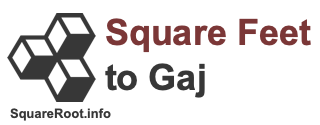Square Feet to Gaj ConverterWelcome to our Square Feet to Gaj Converter. Before we continue, note that this Square Feet to Gaj Converter is the same as sq ft to gaj Converter and ft2 to gaj Converter.

Square feet and gaj are both measurements of an area, like a plot of land. One square foot is equal to 0.112188212713609 gaj. Therefore, we can make the following square feet to gaj formula:

square feet × 0.112188212713609
= gaj

No need to take out your calculator, because we have created the Square Feet to Gaj Converter below. Please enter your area in square feet below to have in converted to gaj.

Convert  square feet to gaj.

Here are some popular square feet to gaj conversions you may find interesting: